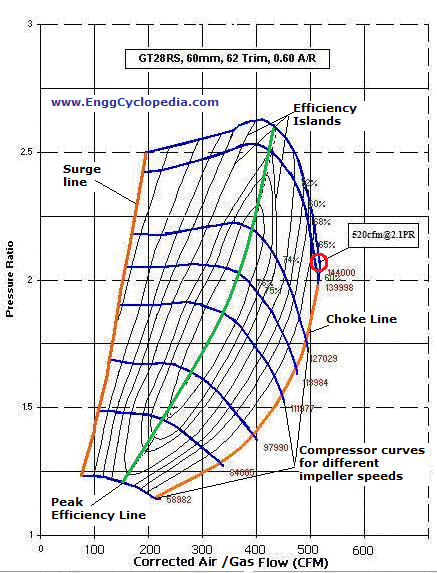# Compressor Maps

## Compressor Maps

Compressor maps are developed by the manufacturer of dynamic compressors. They are compressor equivalents of the pump performance curves.  It is the performance chart of a specific compressor which manufacturer calculates and draws up for the unique design characteristics of that compressor. An example of an air compressor from a car turbocharger is shown in figure – 1.##### Figure 1: Sample air compressor map

Compressor maps are two dimensional and has all the information an engineer needs for design purposes.  In the sample compressor map represented in figure-1, the blue curved lines represent compressor curves for different impeller speed values with the uppermost line being the maximum speed that the compressor can reach. The skewed ellipses are the efficiency “islands” or the efficiency areas. The Y axis is the pressure ratio which is explained below and the X axis is the “air / gas flow before the turbo”. To read of a point from the compressor map is straight forward. For example the red circled point on the map, represents compressor output of 520 CFM (corrected air flow) at a pressure ratio of 2.1. At this point the compressor is spinning at 144000 rpm and has an efficiency of only 61% (indicated by the corresponding efficiency area).

## The Y axis (Vertical Axis)

Y axis of a compressor maps indicates pressure ratio. Pressure ratio is the ratio of the compressor discharge pressure to the compressor suction pressure.  If the compressor suction pressure is known, pressure ratio can be decided to achieve required output. For example if the inlet pressure is 1bar (Atmospheric pressure) and it is required to boost the gas to a pressure of 2 bar, then the pressure ratio needs to be two. The same principal applies to industrial compressors except that they have higher pressure ratios, especially multi-stage compressors. The following is a formula of the calculation that has just been described:

Outlet pressure = Inlet pressure × pressure ratio

Thus if at sea level a certain compressor gives an outlet pressure of 2 bar and then you take the compressor to a much higher elevation the outlet pressure will be lower because the inlet pressure has dropped.

## Efficiency Islands / Efficiency Areas

The “ellipses” can be used just like contours on geographical maps, except that here they show a range of efficiencies. Usually the efficiency islands converge to the center of the compressor map as shown in figure-1, where the efficiency is at its maximum. This line where the efficiency islands on compressor maps converge is known as “Peak Efficiency Line”. Usually operating the compressor near “Peak Efficiency Line” is always the most desirable as the most possible work output can be obtained using same or less work input.

## Surge and Choke lines (Orange)

The orange line on the left hand side is the Surge line and the Orange line on the right hand side is the choke line. If the compressor operates on the left of the surge line, this can result in compressor surge. Compressor surge is a pulsating back flow of gas through the device. Compressor surge is a highly undesirable phenomenon as it can mechanically damage compressor parts and must be avoided.

If the compressor operates on the right of the choke line then the compressor will experience choked flow. Choked flow is when the flow reaches the speed of sound and this is a problem because it limits the maximum flow rate through the compressor. Thus the choke line on a compressor map signifies its maximum flow rate limit. When designing a compressor system careful consideration needs to be taken to make sure that the designed operating point does not fall outside the surge and choke lines.

## Max Flow and Max Pressure

The maximum flow that a compressor can handle is easily found on the sample compressors map, in figure-1. The point of maximum flow is the extreme right point on the map. The red circled point in figure-1 happens to be the point of maximum flow. If more flow needs to be pushed through then a different compressor is required. At the maximum flow point, compressor efficiency is at its lowest so it is highly desirable to use a different compressor.

The maximum discharge pressure that a compressor can achieve is found using the uppermost point on the map. At this point the pressure ratio can be found and using the formula above the outlet pressure can be calculated.

Compressor maps are very important in design of systems because they give you vital information on surge, choke and compressor speeds. They also let you know if your compressor is going to be efficient enough for your application.